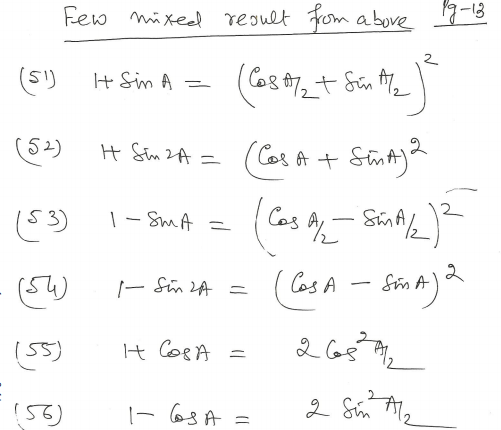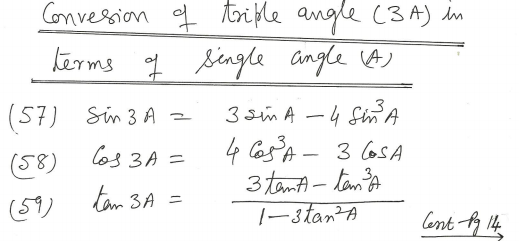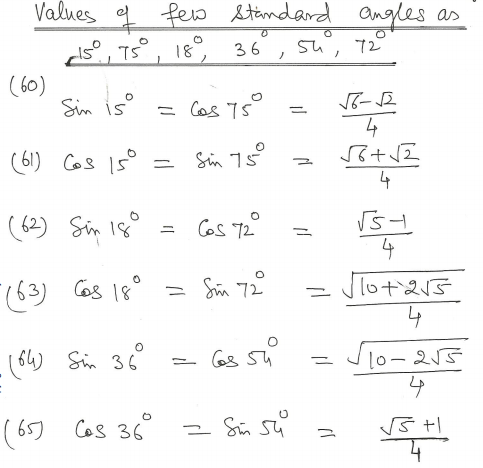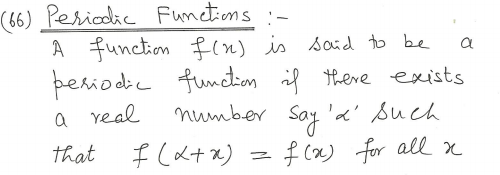# CBSE Class 11 Mathematics Trigonometry Notes Set D

Download CBSE Class 11 Mathematics Trigonometry Notes Set D in PDF format. All Revision notes for Class 11 Mathematics have been designed as per the latest syllabus and updated chapters given in your textbook for Mathematics in Standard 11. Our teachers have designed these concept notes for the benefit of Grade 11 students. You should use these chapter wise notes for revision on daily basis. These study notes can also be used for learning each chapter and its important and difficult topics or revision just before your exams to help you get better scores in upcoming examinations, You can also use Printable notes for Class 11 Mathematics for faster revision of difficult topics and get higher rank. After reading these notes also refer to MCQ questions for Class 11 Mathematics given our website

## Revision Notes for Class 11 Mathematics Trigonometry

Class 11 Mathematics students should refer to the following concepts and notes for Trigonometry in standard 11. These exam notes for Grade 11 Mathematics will be very useful for upcoming class tests and examinations and help you to score good marks

### Trigonometry Notes Class 11 Mathematics## Books recommended by teachers

 CBSE Class 11 Mathematics Set Theory Notes
 CBSE Class 11 Mathematics Relations And Functions Notes
 CBSE Class 11 Mathematics Complex Numbers Quadratic Equations Notes
 CBSE Class 11 Mathematics Linear Inequalities Notes
 CBSE Class 11 Mathematics Permutation And Combination Formulas And Questions CBSE Class 11 Mathematics Permutation And Combination Notes
 CBSE Class 11 Mathematics Sequence And Series Formulas And Questions CBSE Class 11 Mathematics Sequence And Series Notes
 CBSE Class 11 Mathematics Straight Lines Formulas And Questions
 CBSE Class 11 Mathematics Coordinate Geometry Formulas And Questions CBSE Class 11 Mathematics Three Dimensional Geometry Notes
 CBSE Class 11 Mathematics Limits And Derivatives Notes
 CBSE Class 11 Mathematics Mathematical Reasoning Notes
 CBSE Class 11 Mathematics Statistics Notes
 CBSE Class 11 Mathematics Probability Notes